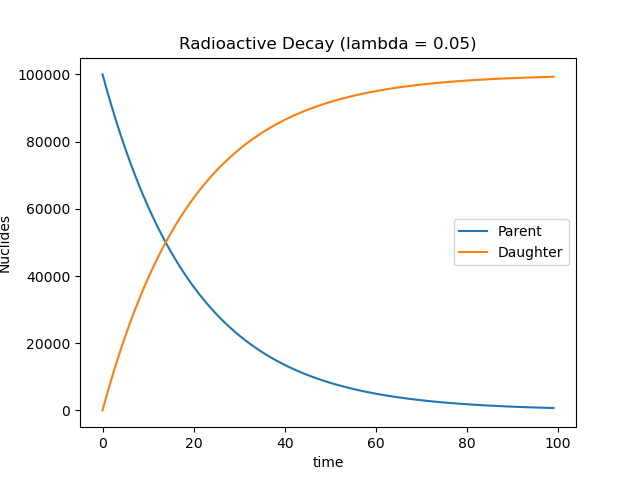When a nuclide is unstable (radioactive) is can decay by various nuclear processes to a lower (stable) energy state. Each nuclide randomly decays following a poisson distribution at a decay rate $$\lambda$$. Since there is a very large number of individual nuclides, we can calculate the average number remaining. Let's assume a radioactive substance decays into a stable daughter element.

$$A^* \rightarrow B$$

The number of radioactive nuclides is $$N$$ and decreases with time as they decay into the daughter nuclude. Thus, $$N=N(t)$$. The rate of change is $$\frac{dN}{dt} = -\lambda \cdot N$$ since the quantity decreases at a constant rate and is proportional to the amount of radioactive nuclides available to decay. Solving the differential equation yields:

$$\frac{dN}{dt} = -\lambda \cdot N$$

$$\int\frac{dN}{N} = \int-\lambda \cdot dt$$

$$ln(N) = -\lambda \cdot t + C$$

$$N(t) = e^{-\lambda \cdot t + C}$$

$$N(t) = e^{-\lambda \cdot t} \cdot e^{C}$$

$$N(t) = e^{-\lambda \cdot t} \cdot C^{'}$$

The initial boundary condition is $$N(t=0)=N_0$$. At time = 0 we have the initial amount of radioactive nucludes $$N_0$$.

$$N(t) = e^{-\lambda \cdot t} \cdot C^{'}$$

$$N(0)=N_0 = e^{-\lambda \cdot (0)} \cdot C^{'}$$

$$N_0 = C^{'}$$

This gives the final equation describing the exponential decay of a radioactive element.

$$N(t) = N_0 \cdot e^{-\lambda \cdot t}$$

There are still $$N_0$$ total nuclide after some time passes but some are now daughter atoms. The number of daughter atoms increases with time:

$$N_D(t) = N_0 - N(t)$$

$$N_D(t) = N_0 - N_0 \cdot e^{-\lambda \cdot t}$$

The relationship between parent and daughter nuclides is shown below.A radioactive sample (10 g) decays for 5 hr. Your lab partner calculates that 3 g of radioactive material remains. What is the mass of the stable nuclide?

## Half-Life

A common quantity in radioactive decay is the half-life. The half-life is the amount of time it takes for half the radioactive substance to decay. After 2 half-lives, 25% of the substance remains and so on. After about 5 half-lives, the radioactive material has mostly decayed.

 # Half-Lives Radioactive Material (%) Stable Material (%) 1 50 50 2 25 75 3 12.5 87.5 4 6.25 93.75 5 3.125 96.875

We can express the formula above in terms of the half-life, $$t_{1/2}$$, accordingly:

$$N(t) = N_0 \cdot e^{-\lambda \cdot t}$$

$$N(t_{1/2})=\frac{N_0}{2} = N_0 \cdot e^{-\lambda \cdot t_{1/2}}$$

$$\frac{N_0}{2} = N_0 \cdot e^{-\lambda \cdot t_{1/2}}$$

$$\frac{1}{2} = e^{-\lambda \cdot t_{1/2}}$$

$$-ln(\frac{1}{2}) = -\lambda \cdot t_{1/2}$$

$$\frac{-ln(\frac{1}{2})}{\lambda} = t_{1/2}$$

$$t_{1/2} = \frac{ln(2)}{\lambda}$$

$$\lambda = \frac{ln(2)}{t_{1/2}}$$

$$N(t) = N_0 \cdot e^{-ln(2) \frac{t}{t_{1/2}}}$$

A radioactive element has a half-life of A mins. If the daughter element is stable, what percentage exists after 3 half-lives.